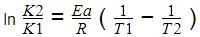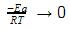Courses

# Test: Temperature Dependence of Reaction

## 10 Questions MCQ Test Chemistry for JEE | Test: Temperature Dependence of Reaction

Description
This mock test of Test: Temperature Dependence of Reaction for JEE helps you for every JEE entrance exam. This contains 10 Multiple Choice Questions for JEE Test: Temperature Dependence of Reaction (mcq) to study with solutions a complete question bank. The solved questions answers in this Test: Temperature Dependence of Reaction quiz give you a good mix of easy questions and tough questions. JEE students definitely take this Test: Temperature Dependence of Reaction exercise for a better result in the exam. You can find other Test: Temperature Dependence of Reaction extra questions, long questions & short questions for JEE on EduRev as well by searching above.
QUESTION: 1

### How many times the rate of reaction increases at 200C for a reaction having the activation energies in the presence and absence of catalyst as 50 kJ/mol and 75 kJ/mol?

Solution:

K = Ae^(-Ea/RT)

Taking ln on both sides

lnk = lnA + (-Ea/R) x 1/T

Now ATQ: lnk1 = lnA + (-Ea1/R) x 1/T

lnk2 = lnA + (-Ea2/R) x 1/T

Subtracting the two equations

ln(k2/k1) = (Ea1 - Ea2)/RT

ln(k2/k1) = (75 - 50) x 1000 / 8.314 x 293

K2/K1 = e (25000 / 8.314 x 293)

K2/K1 = e10.26

This is approximately equal to 30000.

Hence C is correct.

QUESTION: 2

### If Ef and Eb are the activation energies of the forward and reverse reactions and the reaction is known to be exothermic, then:

Solution:

The correct answer is Option B.

For exothermic reaction, ΔH<0
Eb = Ef + ∣ΔH∣ or
Ef < Eb

QUESTION: 3

### The ratio of the rate constant of a reaction at two temperatures differing by __________0C is called temperature coefficient of reaction.

Solution:

The ratio of the rate constant of a reaction at two temperatures differing by 100C is called temperature coefficient of reaction.

QUESTION: 4

The effect of temperature on reaction rate is given by

Solution:
QUESTION: 5

The activation energies of two reactions are given as Ea1= 40 J and Ea2= 80 J, then the relation between their rate constants can be written as:

Solution:

The correct answer is Option A.

As the value of activation energy, Ea increases, the value of rate constant, k decreases.

So, k1 > k2 since E1 < E2

QUESTION: 6

The activation energy of a chemical reaction can be determined by

Solution:

The correct answer is Option C
Activation energy can be determined by evaluation rate constants at different temperatures using equation:Where, K2 = rate constant at temperature T2
K1= rate constant at temperature T1

QUESTION: 7

The rate constant, activation energy and Arrhenius parameter of a chemical reaction at 25°C are 3.0 x 10-4 s-1, 104.4 kJ mol-1 and 6.0 x 1014 s-1 respectively. The value of the rate constant at infinite temperature is is

Solution:

The correct answer is option C
Arrhenius equation
⇒ K = Ae−Ea/RT
As T → ∞,
RT → ∞e−Ea/RT  → 1
Hence K → A as T→∞
∴ Value of K as T → ∞ = 6.0×1014S−1

QUESTION: 8

The plot of ln k vs 1/T is a straight line. The slope of the graph is:

Solution:

The Arrhenius equation is k=Ae−Ea​/RT

ln k = ln A - Ea/RT

comparing with y = mx+c where y = ln k and x = 1/T, slope m becomes -Ea/R

so when ln k vs 1/T is plotted, the slope comes out to be - Ea/R

QUESTION: 9

For a chemical reaction the rate constant is nearly doubled with the rise in temperature by

Solution:

Rate constant is doubled with 10 degree rise in temperature.

QUESTION: 10

The reactions with low activation energy are

Solution:

The correct answer is Option C.

The reactions with low activation energies are always fast whereas the reactions with high activation energy are always slow. The process of speeding up a reaction by reducing its activation energy is known as catalysis, and the factor that's added to lower the activation energy is called a catalyst.